Working with Parabolas

 Solve the following problem involving a parabola: a.  Graph the parabola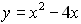on the interval [-1, 5]. b.  Find the turning point (the vertex). c.  Find the axis of symmetry. d.  Find the roots.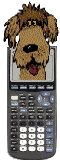a.  To graph the parabola, enter the equation into y =.   The graph can be seen by using a standard 10 x 10 window (Zoom 6), but this question specifies the interval [-1, 5].

 Standard 10 x10 window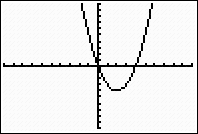Setting WINDOW to [-1, 5]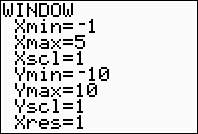Interval [-1, 5]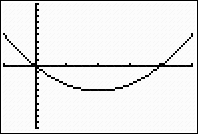b.  To find the turning point, you are looking for the minimum (or maximum) value of the parabola.  For this problem, we will need the minimum option.

Graph the parabola.
 You may be tempted to use the TRACE option to move to the turning point, but be careful.  Used in this manner, the TRACE option may only place you "close" to the turning point and not at its "exact" location.  To be sure you have the "exact" location, use the minimum and maximum options.
Locate minimum
(2nd CALC #3).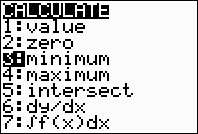For the "left bound", choose any location to the left of the turning point.  Press ENTER.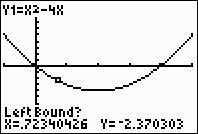An anchor for the left position will appear.  For the "right bound", choose any location to the right of the turning point.  Press ENTER.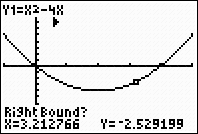Press ENTER to by-pass "guess".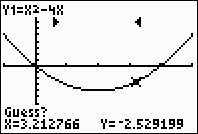The minimum coordinates will appear.  These are the coordinates of the turning point, (2, -4).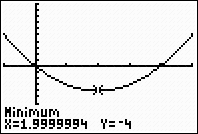Remember, when  you see an answer such as 1.9999994, the actual answer is most likely 2.  To verify, check the value x = 2 into the equation and the result will be y = -4.
 c.  Once you know the turning point, you know where the line which is the axis of symmetry is located.  The axis of symmetry passes through the turning point.  For this example, the axis of symmetry is x = 2.  There is no need to graph the axis of symmetry on the calculator.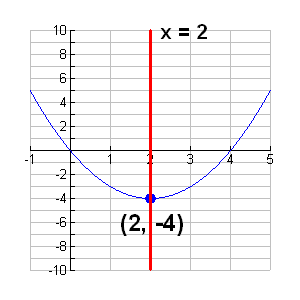d.  To find the roots, you need to find the points at which the graph crosses the x-axis.  Again, do not be tempted to use the TRACE option as it may not give the "exact" locations.  Use the ZERO command to find the roots.

 Locate the zero command. 2nd TRACE (CALC), #2 zero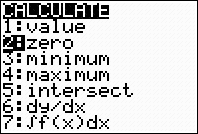Left bound?  Move the spider as close to the root (where the graph crosses the x-axis) as possible.  Now, hit the left arrow again to move to the "left" of the root.  Hit ENTER.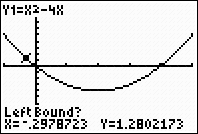A "marker" (anchor) ► will be set to the left of the root. Right bound?  Move the spider as close to the root (where the graph crosses the x-axis) as possible.  Now move to the "right" of the root.  Hit ENTER.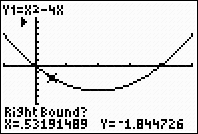A "marker" (anchor) ◄ will be set to the right of the root. Guess?  Just hit ENTER.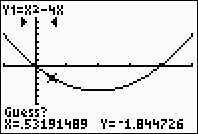The coordinates of the zero  (the root) will appear. Root at x = 0.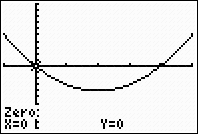Repeat the entire process to find the second root (which in this case happens to be x = 4).Finding Your Way Around TABLE of  CONTENTS